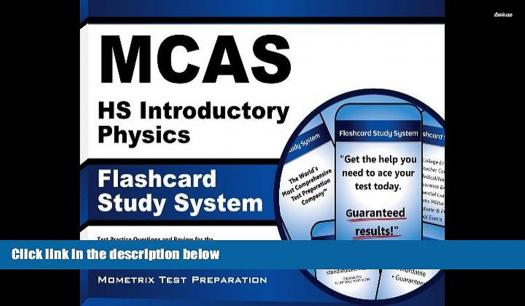# MCAS Physics Exam Practice

10 Questions | Total Attempts: 127SettingsPhysics is a natural science that deals with the study of matter and its motion through space and time. Also it is the study of force of natural world. This study is required as study in which its application is broadly used in all industry and give pace to the high rate of technology in this age.

• 1.
Which of these is not a type of motion?
• A.

Reverse motion

• B.

Rotational motion

• C.

Random motion

• D.

Transnational motion

• 2.
What is the product of mass and velocity?
• A.

Impulse

• B.

Momentum

• C.

Velocity

• D.

Inertia

• 3.
What is the unit of mass?
• A.

Second

• B.

Meter

• C.

Kilogram

• D.

Newton

• 4.
How many seconds make a minute?
• A.

30 seconds

• B.

120 seconds

• C.

45 seconds

• D.

60 seconds

• 5.
Which of these is not a vector quantity?
• A.

Mass

• B.

Force

• C.

Velocity

• D.

Momentum

• 6.
How many grams is in a kilogram?
• A.

0.1

• B.

10

• C.

1000

• D.

100

• 7.
Which of these is not a fundamental quantity?
• A.

Velocity

• B.

Mass

• C.

Time

• D.

Luminous Intensity

• 8.
What is the product of mass and acceleration?
• A.

Displacement

• B.

Impulse

• C.

Velocity

• D.

Force

• 9.
What is motion exhibited by the movement of simple pendulum?
• A.

Translational motion

• B.

Oscillatory motion

• C.

Random motion

• D.

Rotational motion

• 10.
What is the ratio of distance with time?
• A.

Momentum

• B.

Impulse

• C.

Velocity

• D.

Speed

Related TopicsBack to top